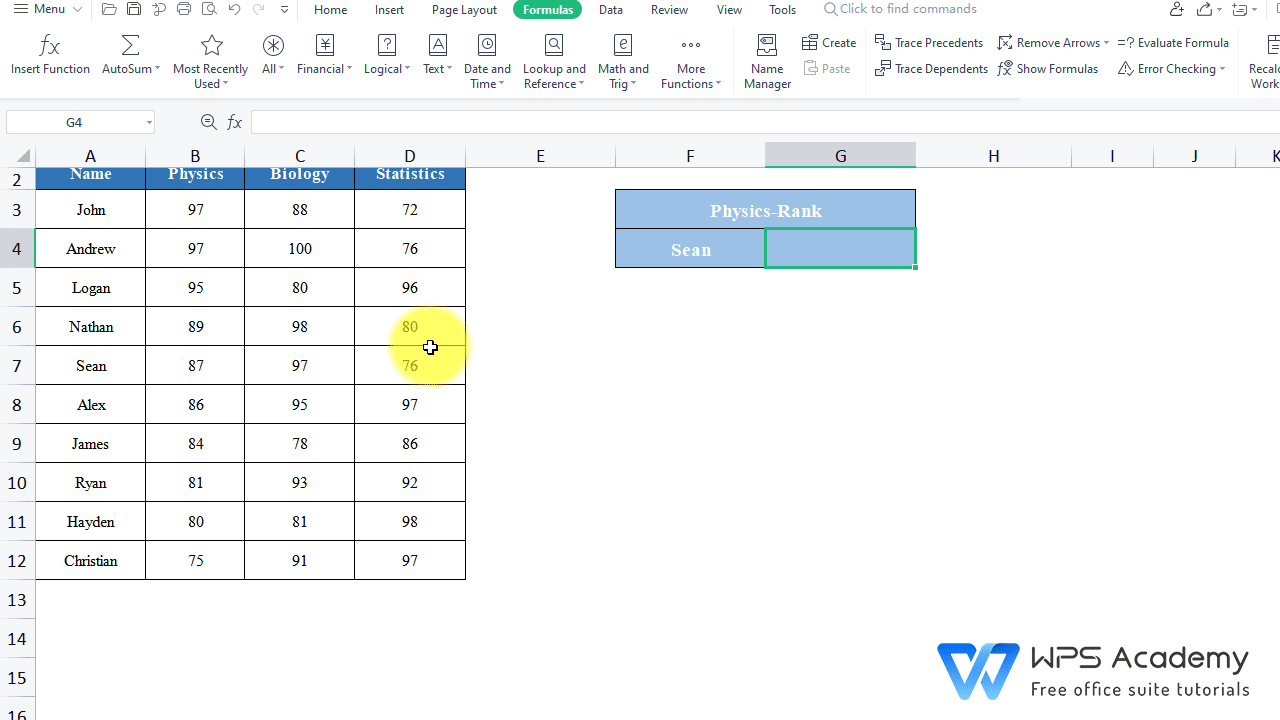# MATCH Function in Excel

December 17, 2021
3.5K Views
0

A free Office suite fully compatible with Microsoft OfficeWPS Spreadsheet could be an alternative to Microsoft Office Excel. It includes 100's of built-in formulas, pivot tables, and more.

· Description:

TheMATCH function can locate the position of a lookup value in which row and column.

· Syntax:

MATCH(lookup_value, lookup_array, [match_type] )

· Arguments:

Lookup_value: the value you want to look up.

Lookup_array:The range of cells that contains the value that you look up.

Match_type: [Optional]. It the type of match that the function will perform.

1: The largest value that is less than or equal to value.

0: The MATCH function will find the first value that is equal to value.

-1: The smallest value that is greater than or equal to value.

· Example:

The physical scores in the table are obviously in descending order. Suppose we want to know Sean's ranking in physics.

2. Head to the Formulas tab > Insert Function > enter MATCH in the edit box.3. In this case, we need to insert MATCH function.

1) Lookup_value is the value you want to look up, we want to locate Sean,  F4 contains his name, so let's enter cell F4 at Lookup_value.

2) Lookup_array is the range of cells that contains the value that you look up. Within this table, Sean's name can be found in the Name column, the Name column is in column A3:A12, so let's enter A3:A12 at Lookup_array.

3) Match_typeis the type of match that the function will perform, which can be omitted if the value is exact. In this case, it is omitted, because we want an exact rank of Sean.

So, we enter the following formula:=MATCH(F4, A3:A12)

Finally, we get a 5,which is to say Sean has the 5th best score in physics.